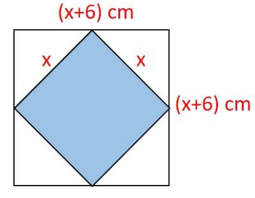Maths-
General
Easy

Question

# What expression represents the total area of the four white triangles?Hint:

## The correct answer is: 12x + 36

### The area of the outer square of side x+6 cm = (x+6)2(x+6)2  = (x+6)(x+6) = x(x+6) +6(x+6)= x(x) + x(6) +6(x) +6(6)= x2 + 6x + 6x + 36= x2 + 12x + 36The area of the inner square of side x cm = x2Now, Total area of four white triangles = Area of the outer square - area of the inner square= x2 + 12x + 36 - x2= 12x + 36Final Answer: Hence, the expression for the total area of the four white triangles is 12x + 36.#### With Turito Foundation.#### Get an Expert Advice From Turito.